Electron. J. Diff. Equ., Vol. 2010(2010), No. 76, pp. 1-9.

### Solutions of a partial differential equation related to the oplus operator Wanchak Satsanit

Abstract: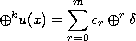where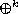is the operator iterated k times and defined by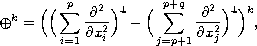where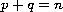,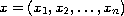is in the n-dimensional Euclidian space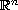,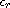is a constant,is the Dirac-delta distribution,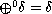, and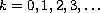. It is shown that, depending on the relationship between k and m, the solution to this equation can be ordinary functions, tempered distributions, or singular distributions.

Submitted April 8, 2010. Published June 8, 2010.
Math Subject Classifications: 46F10, 46F12.
Key Words: Ultra-hyperbolic kernel; diamond operator; tempered distribution.

Show me the PDF file (213 KB), TEX file, and other files for this article.Wanchak Satsanit Department of Mathematics Faculty of Science, Maejo University Chiang Mai, 50290 Thailand email: aunphue@live.com